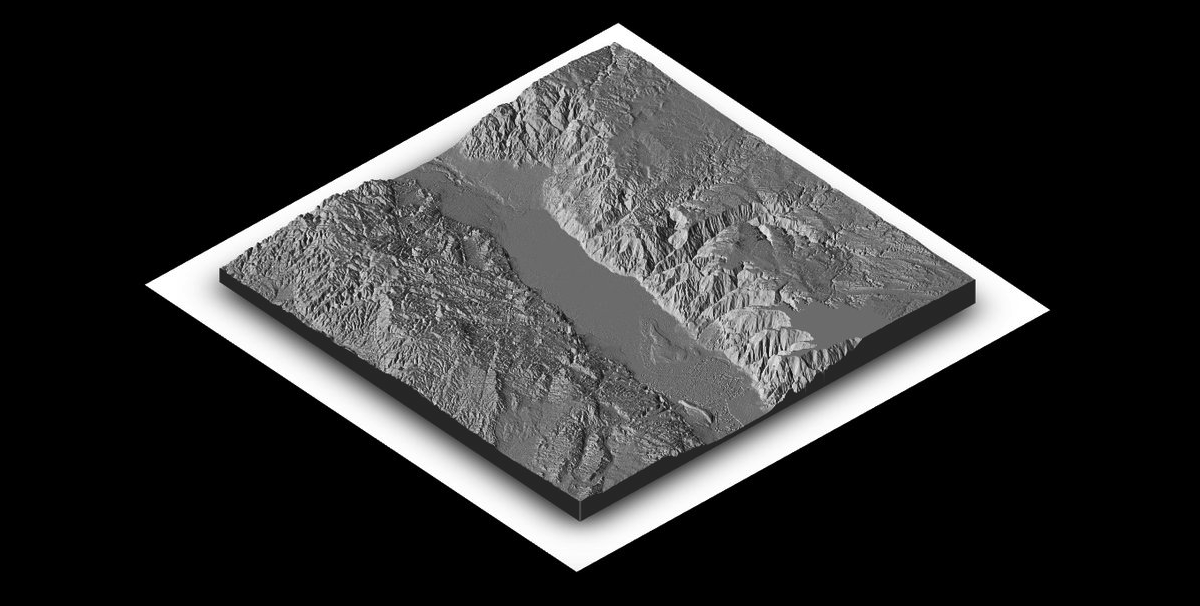``````
# devtools::install_github("tylermorganwall/rayshader")
library(rayshader)
library(raster)

# quick function to extract a matrix from the raw raster
matrix_extract <- function(raw_raster) {
matrix(raster::extract(raw_raster, extent(raw_raster), buffer = 1000),
nrow = ncol(raw_raster),
ncol = nrow(raw_raster))

}

# import raster
# original file is N31E035 from the USGS respository https://dds.cr.usgs.gov/srtm/version1/Africa/
dead_sea <- raster("dead_sea.tif")

# extract the matrix for ploting
dead_sea_matrix <- matrix_extract(dead_sea)

dead_sea_matrix %>%
sphere_shade(texture= "bw") %>%
# juicing this a bit where with zscale = 4
plot_3d(dead_sea_matrix, solid = T, shadow = T, zscale = 4, background = "black")

``````